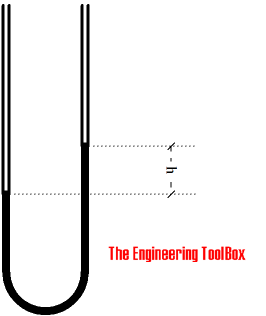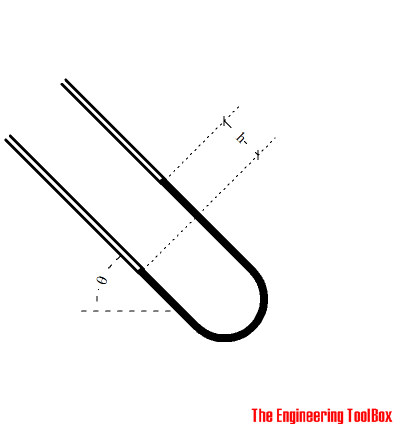Engineering ToolBox - Resources, Tools and Basic Information for Engineering and Design of Technical Applications!

# U-Tube Differential Pressure Manometers

## Inclined and vertical u-tube manometers used to measure differential pressure in flow meters like pitot tubes, orifices and nozzles.

Pressure measuring devices using liquid columns in vertical or inclined tubes are called manometers. One of the most common is the water filled u-tube manometer used to measure pressure difference in pitot or orifices located in the airflow in air handling or ventilation system.

In the figure bellow illustrates the water levels in an u-tube where the left tube is connected to a point with higher pressure than the right tube - example: the left tube may be connected to a pressurized air duct when the right tube is open to the ambient air.### Vertical U-Tube Manometer

The pressure difference measured by a vertical U-Tube manometer can be calculated as

pd = γ h

= ρ g h                                                (1)

where

pd = pressure (Pa, N/m2, lb/ft2)

γ = ρ g = specific weight of liquid in the tube (kN/m3, lb/ft3 )

ρ = U-tube liquid density (kg/m3, lb/ft3)

g = acceleration of gravity (9.81 m/s2, 32.174 ft/s2)

h = liquid height (m fluid column, ft fluid column)

The specific weight of water, which is the most commonly used fluid in u-tube manometers, is 9.81 kN/m3 or 62.4 lb/ft3.

Note! - the head unit is with reference to the density of the flowing fluid. For other units and reference liquid - like mm Water Column - check Velocity Pressure Head.

#### Example - Orifice Differential Pressure Measurement

A water manometer connects the upstream and downstream pressure of an orifice located in an air flow. The difference height in the water column is 10 mm.

The pressure difference head can calculated from (1) as

pd = (9.8 kN/m3) (103 N/kN) (10 mm) (10-3 m/mm)

= 98 (N/m2, Pa)

where

9.8 (kN/m3) is the specific weight of water in SI-units.

### Inclined U-Tube Manometer

A common problem when measuring the pressure difference in low velocity systems - or systems with low density fluids - like air ventilation systems - are low column heights and accuracy. Accuracy can be improved by inclining the u-tube manometer.

The figure bellow indicates a u-tube where the left tube is connected to a higher pressure than the right tube. Note that the left and the right tube must in the same declined plane for the angle to the horizontal plane to be correct.The pressure difference in a inclined u-tube manometer can be expressed as

pd = γ h sin(θ)                                               (2)

where

h = length, difference in position of the liquid column along the tube (mm, ft)

θ = angle of column relative the horizontal plane (degrees)

Inclining the tube manometer increases the accuracy of the measurement.

#### Example - Differential Pressure Measurement with an Inclined U-Tube manometer

We use the same data as in the example above, except that the U-Tube is inclined 45o.

The pressure difference head can then be expressed as:

pd = (9.8 kN/m3) (103 N/kN) (10 mm) (10-3 m/mm) sin(45o)

= 69.3 N/m2 (Pa)

### U-tube Manometer Calculator

This calculator can be used to calculate the differential pressure measured with an U-tube manometer.

γ - specific weight of the fluid in the tube (kN/m3, lb/ft3 )

(9.8 kN/m3, 62.4 lb/ft3 default values for water)

h - length of the liquid column along the tube (mm, ft)

θ - angle of column relative the horizontal plane (degrees)

SIImperial

## Related Topics

### • Flow Measurement

Flow metering principles - Orifice, Venturi, Flow Nozzles, Pitot Tubes, Target, Variable Area, Positive Displacement, Turbine, Vortex, Electromagnetic, Ultrasonic Doppler, Ultrasonic Time-of-travel, Mass Coriolis, Mass Thermal, Weir V-notch, Flume Parshall and Sluice Gate flow meters and more.

### • Fluid Mechanics

The study of fluids - liquids and gases. Involving velocity, pressure, density and temperature as functions of space and time.

### • Ventilation

Systems for ventilation and air handling - air change rates, ducts and pressure drops, charts and diagrams and more.

## Engineering ToolBox - SketchUp Extension - Online 3D modeling!

Add standard and customized parametric components - like flange beams, lumbers, piping, stairs and more - to your Sketchup model with the Engineering ToolBox - SketchUp Extension - enabled for use with the amazing, fun and free SketchUp Make and SketchUp Pro . Add the Engineering ToolBox extension to your SketchUp from the SketchUp Pro Sketchup Extension Warehouse!

Translate

## Privacy

We don't collect information from our users. Only emails and answers are saved in our archive. Cookies are only used in the browser to improve user experience.

Some of our calculators and applications let you save application data to your local computer. These applications will - due to browser restrictions - send data between your browser and our server. We don't save this data.